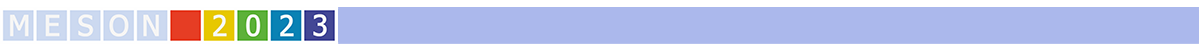#MESON2023

Jun 22 – 27, 2023
Auditorium Maximum
Europe/Warsaw timezone

## The reaction $\pi N \to \omega N$ in a dynamical coupled-channel approach

Jun 26, 2023, 3:40 PM
20m
Medium lecture hall (A) (Auditorium Maximum)

Parallel

### Speaker

Yu-Fei Wang (Forschungszentrum Jülich)

### Description

This talk is on a refined investigation on light flavor meson-baryon scatterings, using a dynamical coupled-channel approach, i.e. the Jülich-Bonn model. The previous channel space of $\pi N$, $\pi \Delta$, $\sigma N$, $\rho N$, $\eta N$, $K \Lambda$ and $K \Sigma$ is extended by adding the $\omega N$ final state. The spectra of $N^*$ and $\Delta$ resonances are extracted, based on the result of a global fit to a worldwide collection of data, in the energy region from the $\pi N$ threshold to center-of-mass energy $z=2.3$ GeV (approximately 300 parameters against 9000 data points). A negative value of the $\omega N$ elastic spin-averaged scattering length has been extracted.

### Primary author

Yu-Fei Wang (Forschungszentrum Jülich)